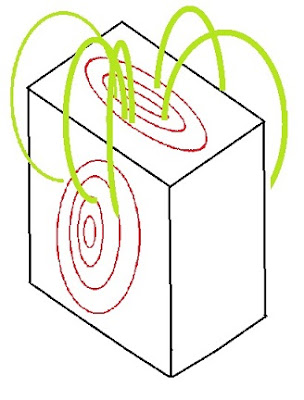## Sunday, February 27, 2011

### Counting Modes

How many ways can you set up a standing mode of electromagnetic waves in a rectangular cavity? This question has been holding me up for a week now. There is a formula which I found on the hyperphysics webpage; it is basically the Rayleigh-Jeans analysis which gives the low-frequency limit of the black-body law. The formula must be right because it agrees with experimental results, assuming each mode gets its alloted energy of 1/2kT. My problem is I have my own way of counting modes which disagrees by a factor of 3, and I can't for the life of me see where.

There are a lot of ways you can be out by a factor of 2 or 4, but it is very hard to be out by a factor of three. Furthermore, I understand the basic concept of counting modes. I know how to do it in two dimensions for electromagnetics, and know how to do it in three dimensions for a gas. I thought I knew how to do it in three dimensions for solids, but now I'm not so sure. At first I thought it was the same as a gas, but now I realize that a solid, unlike a gas, can support shear waves as well as compressional waves.

The electromagnetic case in two dimensions is not so bad because you can start by taking waves in the xy plane and letting the polarization be all in the z direction. That lets you separate out the vector portion and leaves you with nothing but scalar waves to add up in the plane. It doesn't take much more than high-school trig identities to show that four sets of plane waves criss-crossing each other can be added up to equal a checkerboard pattern of standing waves.

That's how the hyperphysics explanation starts out, except it's in three dimensions. Here is what they say:I really don't see that this can be correct. They call for the electric field to go to zero at the cavity walls, which is of course the correct condition for acoustic vibration amplitude in a gas. But electromagnetics? It's certainly not the condition for a waveguide. We need the electric field to have no tangential component, and we need the magnetic field to have no perpendicular component at the walls. Those are very different conditions than what they've described here. But never mind, let's go on.
We now have the rectangular cavity divided up into unit cells, and we want to count the modes up to a given wavelength. They do the thing of working in k-vector space and counting the lattice points within a spherical volume. I haven't explained it in detail but it's the same thing I do and they end up with the following formula:It's essentially the number of unit cells, with an extra factor of two for what they say is polarization. I think that's also wrong; I would say the factor of two is necessary for the time displacement of one-quarter cycle; but that's a technical point. In any case I said I wouldn't be arguing about factors of two. It's the factor of three that's getting me.

What exactly does the electromagnetic field look like?? I first thought that it could be polarized in either the x, y, or z directions, giving me an extra factor of three. But then I realized that didn't make sense. In two dimensions you can add up all kinds of waves in the xy plane, keeping the polarization in the z plane. But there's no way you can take plane waves in three dimensions and add them up with all the same polarization. The polarizations is going to vary in different places... in other words, the field lines are going to curve.

How can you picture field lines curving through space and still meeting the required boundary conditions? Not the simplistic, incorrect boundary conditions described in the hyperphysics, link, but the correct e-m cavity conditions: perfectly conducting walls with the electric field perpendicular and the magnetic field tangential? After some hours staring at a box of Rice Krispies (as my typical rectangular cell) and drawing ellipses on different faces, this is what I came up with:The green lines are the electric fields and the red ellipses are the magnetic fields. I am a bit troubled by a certain assymetry .... the unit cell has two "ellipsoids" of magnetic lines and one of electrical lines. (I've drawn the green electrical lines as though they form a torus, but that's bad artwork: the ellipses should be parallel.) Still, I think this is the correct configuration. It seems to work: as the magnetic loops collapse, the generate electric loops in generally the right places.

It's the count that bothers me. Of the six cell walls, four have magnetic loops on them and two are empty... in this case the side walls (the walls where they list the ingredients on the Rice Krispies box.) But I could have just as easily taken the front/back or the top/bottom faces to be the null faces. So there are three field configurations that fit this geometry. I can't think of any physical reason that shouldn't work.

So my mode count disagrees with Rayleigh-Jeans by a factor of three. And yet the Rayleigh-Jeans formula gives the correct energy for the field (at low frequencies), assuming each mode gets its alloted energy. It's a problem and I can't get around it.

For now I think I'm going to make use of the Rayleigh-Jeans formula and go ahead with my equilibrium calculations.

JAN 24 2013: It's taken me almost two years, but I found the flaw in my counting method. You can see what I did wrong if you click here.